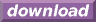#Calcute: freeware calculator

## Hyperbolic Arccosine (hyperbolic arc-cosine, hyperbolic inverse cosine, acosh)

The hyperbolic arc-cosine is the inverse of the hyperbolic cosine function. Calcute implements it with the acosh function. The inverse hyperbolic cosine of a value x is the value y for which the hyperbolic cosine of y is x. In other words if y = acosh(x) then x = cosh(y).

```acosh(1)
0
```

The hyperbolic arc-cosine function is only defined for parameter values of +1 and higher, which corresponds to the output range of the hyperbolic cosine function. Note that the hyperbolic cosine is symmetrical across the Y axis: the hyperbolic cosine of an argument is the same as for the negative value of the same argument. Conversely, the hyperbolic arccosine is a bi-valued function: any parameter in the valid range matches both a positive and a negative number. But the acosh function generates a single (principal) value: the positive one. The other, negative possibility is obtained by negating the return value of acosh.

In some mathematical texts and hand-held calculators, the notation cosh-1 is sometimes used to represent the hyperbolic arc-cosine function. This is an unfortunate notational choice since it could also stand for the multiplicative inverse 1/cosh, which is a different function.

Yet another term also used to represent the inverse hyperbolic cosine function is "arccosh".